## ใครมี Function อ่านเงินบาท เป็นภาษาอังกฤษ ขอหน่อยครับ

0 สมาชิก และ 1 บุคคลทั่วไป กำลังดูหัวข้อนี้

25 ก.พ. 63 , 11:04:12
อ่าน 577 ครั้ง

#### Krathok-man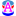##### ใครมี Function อ่านเงินบาท เป็นภาษาอังกฤษ ขอหน่อยครับ
« เมื่อ: 25 ก.พ. 63 , 11:04:12 »
เช่น  65.00 อ่านว่า "sixty five bath"  เป็นต้น จนถึงหลักล้าน

25 ก.พ. 63 , 14:39:57
ตอบกลับ #1

#### PNR

• Programer สาย DIY
•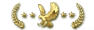• กระทู้: 823
• พลังขอบคุณ: 704
•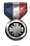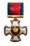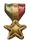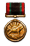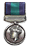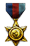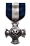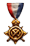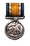• Cooperative Learning##### : ใครมี Function อ่านเงินบาท เป็นภาษาอังกฤษ ขอหน่อยครับ
« ตอบกลับ #1 เมื่อ: 25 ก.พ. 63 , 14:39:57 »
เช่น  65.00 อ่านว่า "sixty five bath"  เป็นต้น จนถึงหลักล้าน

สร้าง Module ใส่โค้ดนี้เข้าไป

โค๊ด: [Select]
`Public Function wsiSpellNumber(ByVal MyNumber)    Dim Bath, Stang, Temp    Dim DecimalPlace, Count    ReDim Place(9) As String    Place(2) = " Thousand "    Place(3) = " Million "    Place(4) = " Billion "    Place(5) = " Trillion "    ' String representation of amount.    MyNumber = Trim(Str(MyNumber))    ' Position of decimal place 0 if none.    DecimalPlace = InStr(MyNumber, ".")    ' Convert Stang and set MyNumber to dollar amount.    If DecimalPlace > 0 Then        Stang = GetTens(Left(Mid(MyNumber, DecimalPlace + 1) & _                  "00", 2))        MyNumber = Trim(Left(MyNumber, DecimalPlace - 1))    End If    Count = 1    Do While MyNumber <> ""        Temp = GetHundreds(Right(MyNumber, 3))        If Temp <> "" Then Bath = Temp & Place(Count) & Bath        If Len(MyNumber) > 3 Then            MyNumber = Left(MyNumber, Len(MyNumber) - 3)        Else            MyNumber = ""        End If        Count = Count + 1    Loop    Select Case Bath        Case ""           Bath = "No Bath"        Case "One"            Bath = "One Bath"         Case Else            Bath = Bath & " Bath"    End Select    Select Case Stang        Case ""            Stang = " and No Stang"        Case "One"            Stang = " and One Stang"              Case Else           Stang = " and " & Stang & " Stang"    End Select    wsiSpellNumber = Bath & StangEnd Function      ' Converts a number from 100-999 into textFunction GetHundreds(ByVal MyNumber)    Dim result As String    If Val(MyNumber) = 0 Then Exit Function    MyNumber = Right("000" & MyNumber, 3)    ' Convert the hundreds place.    If Mid(MyNumber, 1, 1) <> "0" Then        result = GetDigit(Mid(MyNumber, 1, 1)) & " Hundred "    End If    ' Convert the tens and ones place.    If Mid(MyNumber, 2, 1) <> "0" Then        result = result & GetTens(Mid(MyNumber, 2))    Else        result = result & GetDigit(Mid(MyNumber, 3))    End If    GetHundreds = resultEnd Function      ' Converts a number from 10 to 99 into text.Function GetTens(TensText)    Dim result As String    result = ""           ' Null out the temporary function value.    If Val(Left(TensText, 1)) = 1 Then   ' If value between 10-19...        Select Case Val(TensText)            Case 10: result = "Ten"            Case 11: result = "Eleven"            Case 12: result = "Twelve"            Case 13: result = "Thirteen"            Case 14: result = "Fourteen"            Case 15: result = "Fifteen"            Case 16: result = "Sixteen"            Case 17: result = "Seventeen"            Case 18: result = "Eighteen"            Case 19: result = "Nineteen"            Case Else        End Select    Else                                 ' If value between 20-99...        Select Case Val(Left(TensText, 1))            Case 2: result = "Twenty "            Case 3: result = "Thirty "            Case 4: result = "Forty "            Case 5: result = "Fifty "            Case 6: result = "Sixty "            Case 7: result = "Seventy "            Case 8: result = "Eighty "            Case 9: result = "Ninety "            Case Else        End Select        result = result & GetDigit _            (Right(TensText, 1))  ' Retrieve ones place.    End If    GetTens = resultEnd Function     ' Converts a number from 1 to 9 into text.Function GetDigit(Digit)    Select Case Val(Digit)        Case 1: GetDigit = "One"        Case 2: GetDigit = "Two"        Case 3: GetDigit = "Three"        Case 4: GetDigit = "Four"        Case 5: GetDigit = "Five"        Case 6: GetDigit = "Six"        Case 7: GetDigit = "Seven"        Case 8: GetDigit = "Eight"        Case 9: GetDigit = "Nine"        Case Else: GetDigit = ""    End SelectEnd Function`
เวลาเรียกใช้ก็ใส่ในคิวรี่ ก็ใส่ EngText:wsiSpellNumber([ชื่อฟิลล์]) หรือ ตัวแปรที่จำให้แสดง

Credit : www.microsoftaccessexpert.com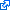« แก้ไขครั้งสุดท้าย: 25 ก.พ. 63 , 14:45:45 โดย PNR »
Time to stop for me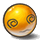โพสต์นี้ได้รับคำขอบคุณจาก: Krathok-man, SakDa

25 ก.พ. 63 , 15:18:14
ตอบกลับ #2

#### Krathok-man##### : ใครมี Function อ่านเงินบาท เป็นภาษาอังกฤษ ขอหน่อยครับ
« ตอบกลับ #2 เมื่อ: 25 ก.พ. 63 , 15:18:14 »
ขอบคุณครับ  ใช้ได้ดีทีเดียว

25 ก.พ. 63 , 16:02:29
ตอบกลับ #3

#### PNR

• Programer สาย DIY
•• กระทู้: 823
• พลังขอบคุณ: 704
•• Cooperative Learning##### : ใครมี Function อ่านเงินบาท เป็นภาษาอังกฤษ ขอหน่อยครับ
« ตอบกลับ #3 เมื่อ: 25 ก.พ. 63 , 16:02:29 »
Update นิดนึงครับ กรณีไม่มีเศษสตางค์ ให้แสดงแค่บาท ไม่มีสตางค์
โค๊ด: [Select]
`Public Function wsiSpellNumber(ByVal MyNumber)    Dim Bath, Stang, Temp    Dim DecimalPlace, Count    ReDim Place(9) As String    Place(2) = " Thousand "    Place(3) = " Million "    Place(4) = " Billion "    Place(5) = " Trillion "    ' String representation of amount.    MyNumber = Trim(Str(MyNumber))    ' Position of decimal place 0 if none.    DecimalPlace = InStr(MyNumber, ".")    ' Convert Stang and set MyNumber to dollar amount.    If DecimalPlace > 0 Then        Stang = GetTens(Left(Mid(MyNumber, DecimalPlace + 1) & _                  "00", 2))        MyNumber = Trim(Left(MyNumber, DecimalPlace - 1))    End If    Count = 1    Do While MyNumber <> ""        Temp = GetHundreds(Right(MyNumber, 3))        If Temp <> "" Then Bath = Temp & Place(Count) & Bath        If Len(MyNumber) > 3 Then            MyNumber = Left(MyNumber, Len(MyNumber) - 3)        Else            MyNumber = ""        End If        Count = Count + 1    Loop    Select Case Bath        Case ""           Bath = ""        Case "One"            Bath = "One Bath"         Case Else            Bath = Bath & " Bath"    End Select    Select Case Stang        Case ""            Stang = ""        Case "One"            Stang = " and One Stang"              Case Else           Stang = " and " & Stang & " Stang"    End Select    wsiSpellNumber = Bath & StangEnd Function      ' Converts a number from 100-999 into textFunction GetHundreds(ByVal MyNumber)    Dim result As String    If Val(MyNumber) = 0 Then Exit Function    MyNumber = Right("000" & MyNumber, 3)    ' Convert the hundreds place.    If Mid(MyNumber, 1, 1) <> "0" Then        result = GetDigit(Mid(MyNumber, 1, 1)) & " Hundred "    End If    ' Convert the tens and ones place.    If Mid(MyNumber, 2, 1) <> "0" Then        result = result & GetTens(Mid(MyNumber, 2))    Else        result = result & GetDigit(Mid(MyNumber, 3))    End If    GetHundreds = resultEnd Function      ' Converts a number from 10 to 99 into text.Function GetTens(TensText)    Dim result As String    result = ""           ' Null out the temporary function value.    If Val(Left(TensText, 1)) = 1 Then   ' If value between 10-19...        Select Case Val(TensText)            Case 10: result = "Ten"            Case 11: result = "Eleven"            Case 12: result = "Twelve"            Case 13: result = "Thirteen"            Case 14: result = "Fourteen"            Case 15: result = "Fifteen"            Case 16: result = "Sixteen"            Case 17: result = "Seventeen"            Case 18: result = "Eighteen"            Case 19: result = "Nineteen"            Case Else        End Select    Else                                 ' If value between 20-99...        Select Case Val(Left(TensText, 1))            Case 2: result = "Twenty "            Case 3: result = "Thirty "            Case 4: result = "Forty "            Case 5: result = "Fifty "            Case 6: result = "Sixty "            Case 7: result = "Seventy "            Case 8: result = "Eighty "            Case 9: result = "Ninety "            Case Else        End Select        result = result & GetDigit _            (Right(TensText, 1))  ' Retrieve ones place.    End If    GetTens = resultEnd Function     ' Converts a number from 1 to 9 into text.Function GetDigit(Digit)    Select Case Val(Digit)        Case 1: GetDigit = "One"        Case 2: GetDigit = "Two"        Case 3: GetDigit = "Three"        Case 4: GetDigit = "Four"        Case 5: GetDigit = "Five"        Case 6: GetDigit = "Six"        Case 7: GetDigit = "Seven"        Case 8: GetDigit = "Eight"        Case 9: GetDigit = "Nine"        Case Else: GetDigit = ""    End SelectEnd Function`
Time to stop for me